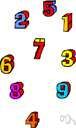# arithmetic progression

(redirected from Arithmetic sequence)
Also found in: Thesaurus, Financial, Encyclopedia.
Related to Arithmetic sequence: Geometric sequence, Arithmetic series

## arithmetic progression

n.
A sequence, such as the positive odd integers 1, 3, 5, 7, ... , in which each term after the first is formed by adding a constant to the preceding term.

## arithmetic progression

n
(Mathematics) a sequence of numbers or quantities, each term of which differs from the succeeding term by a constant amount, such as 3,6,9,12. Compare geometric progression

## arithmet′ic progres′sion

n.
a sequence in which each term is obtained by the addition of a constant number to the preceding term, as 1, 4, 7, 10, and 6, 1, −4, −9. Also called ar′ithmet′ic se′ries.

## ar·ith·met·ic progression

(ăr′ĭth-mĕt′ĭk)
A sequence of numbers such as 1, 3, 5, 7, 9 ..., in which each term after the first is formed by adding a constant to the preceding number (in this case, 2). Compare geometric progression.

## arithmetic progression

- A sequence in which each term is obtained by the addition of a constant number to the preceding term, as 1, 4, 7, 10, 13.
 Noun 1arithmetic progression - (mathematics) a progression in which a constant is added to each term in order to obtain the next term; "1-4-7-10-13- is the start of an arithmetic progression"math, mathematics, maths - a science (or group of related sciences) dealing with the logic of quantity and shape and arrangementpatterned advance, progression - a series with a definite pattern of advance